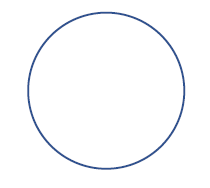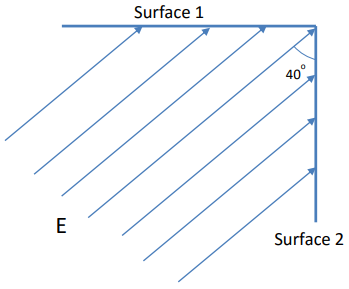Clutch Prep is now a part of Pearson
Ch 25: Electric Force & Field; Gauss' LawWorksheetSee all chapters

# Electric Flux

See all sections
Sections
Electric Charge
Charging Objects
Charging By Induction
Conservation of Charge
Coulomb's Law (Electric Force)
Electric Field
Electric Fields in Capacitors
Electric Field Lines
Dipole Moment
Electric Fields in Conductors
Electric Flux
Gauss' Law

Concept #1: Electric Flux

Practice: The electric flux through each surface of a cube is given below. Which surfaces of the cube does the electric field run parallel to?

Φ1 = 100 Nm2 /C           Φ4 = 0 Nm2 /C
Φ2 = 20 Nm2 /C             Φ5 = −40 Nm2 /𝐶
Φ3 = 0 Nm2 /C               Φ6 = −80 Nm2 /𝐶

Example #1: Flux Through Angled Surface

Example #2: Flux Through Cube

Practice: Where does the normal vector point for a spherical shell?Example #3: Flux Through Spherical Shell due to Point Charge

Practice: What is the total flux through the two surfaces depicted in the following figure? Note that surface 1 has an area of 50 cm2 and surface 2 has an area of 100 cm2 , and E = 500 N/C.